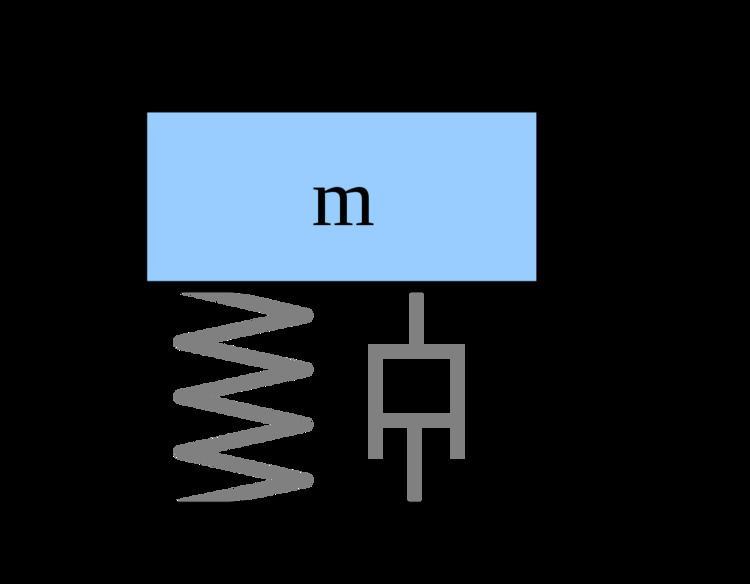# Eigensystem realization algorithm

Updated onThe Eigensystem realization algorithm (ERA) is a system identification technique popular in civil engineering, in particular in structural health monitoring. ERA can be used as a modal analysis technique and generates a system realization using the time domain response (multi-)input and (multi-)output data. The ERA was proposed by Juang and Pappa and has been used for system identification of aerospace structures such as the Galileo spacecraft, turbines, civil structures and many other type of systems.

## Uses in structural engineering

In structural engineering the ERA is used to identify natural frequencies, mode shapes and damping ratios. The ERA is commonly used in conjunction with the Natural Excitation Technique (NExT) to identify modal parameters from ambient vibration. The technique has been applied to buildings, bridges, and many other type of structural systems. In the area of structural health monitoring the ERA and other modal identification techniques play an important role in developing a model of the structure from experimental data. The state space representation, or the modal parameters are used for further analysis and identify possible damage in structures.

## Algorithm

It is recommended to review the concepts of State-space representation and vibration before studying the ERA. Given pulse response data form the Hankel matrix

H ( k 1 ) = [ Y ( k ) Y ( k + 1 ) Y ( k + p ) Y ( k + 1 ) Y ( k + r ) Y ( k + p + r ) ]

where Y ( k ) is the m × n pulse response at time step k . Next, perform a singular value decomposition of H ( 0 ) , i.e. H ( 0 ) = P D Q T . Then choose only the rows and columns corresponding to physical modes to form the matrices D n , P n ,  and  Q n . Then the discrete time system realization can be given by:

A ^ = D n 1 2 P n T H ( 1 ) Q n D n 1 2 B ^ = D n 1 2 Q n T E m C ^ = E n T P n D n 1 2

To generate the system states Λ = C ^ Φ ^ where Φ ^ is the matrix of eigenvectors for A ^ .

## Example

Consider a single degree of freedom (SDOF) system with stiffness k , mass m , and damping c . The equation of motion for this SDOF is

m x ¨ ( t ) + c x ˙ ( t ) + k x ( t ) = p ( t )

where x is the displacement of the mass and t is time. The continuous state-space representation of this system is

s ˙ = A s ( t ) + B u ( t ) y = C s ( t ) + D u ( t )

where s represent the states of the system corresponding to the displacement x and velocity x ˙ of the SDOF. Note that the states are usually denoted by x . However, here x is used for the SDOF displacement.

## References

Similar Topics
Sakkarai Devan
Alberto Lopez Fernandez
Quentin Ngakoutou
Topics